# Cumulative Frequency Graphs Card Match In this activity

• Slides: 16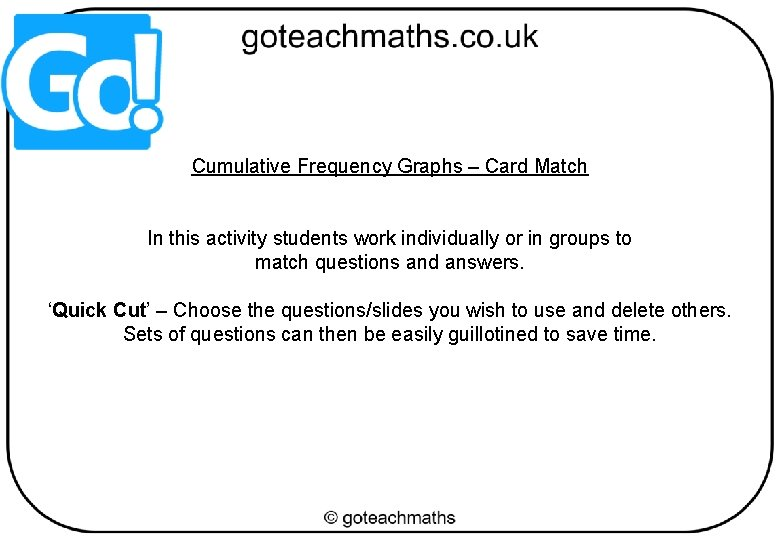Cumulative Frequency Graphs – Card Match In this activity students work individually or in groups to match questions and answers. ‘Quick Cut’ – Choose the questions/slides you wish to use and delete others. Sets of questions can then be easily guillotined to save time.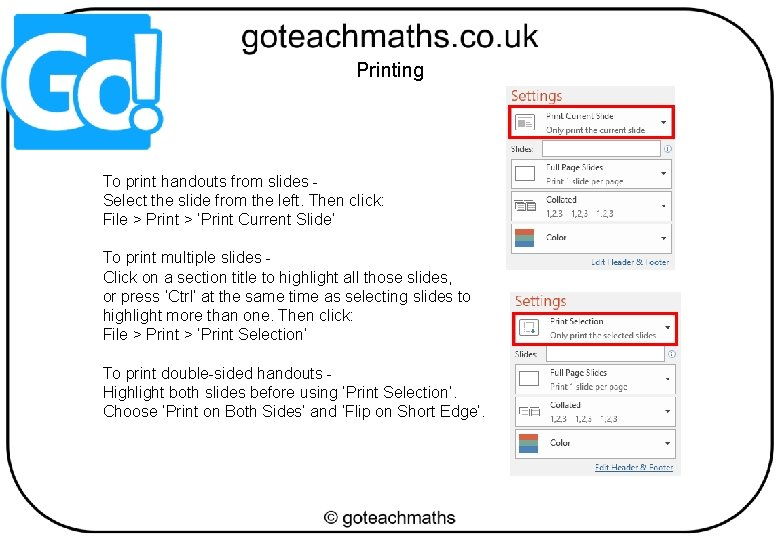Printing To print handouts from slides Select the slide from the left. Then click: File > Print > ‘Print Current Slide’ To print multiple slides Click on a section title to highlight all those slides, or press ‘Ctrl’ at the same time as selecting slides to highlight more than one. Then click: File > Print > ‘Print Selection’ To print double-sided handouts Highlight both slides before using ‘Print Selection’. Choose ‘Print on Both Sides’ and ‘Flip on Short Edge’.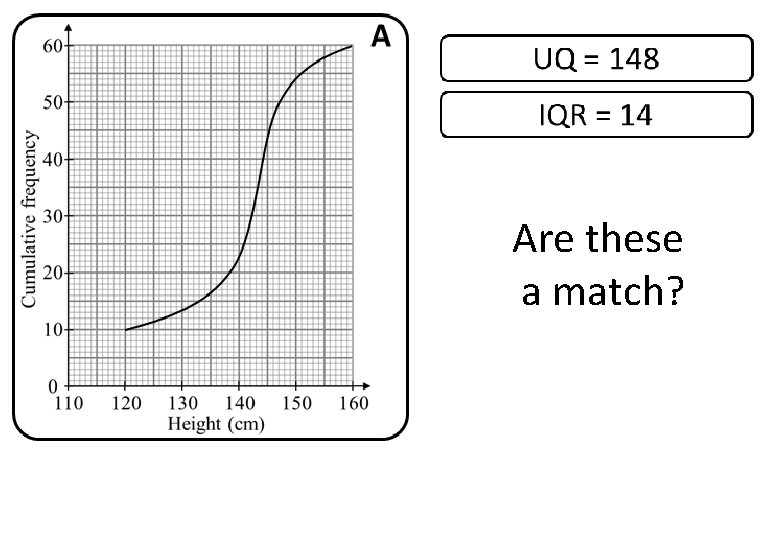Are these a match?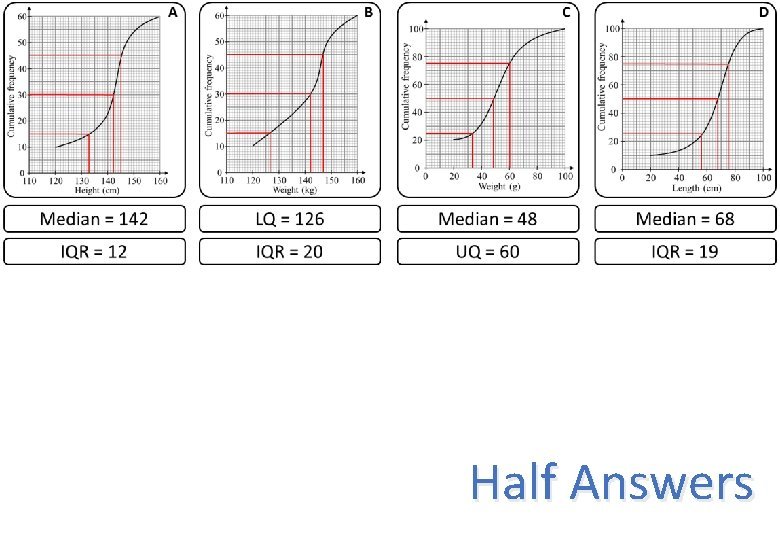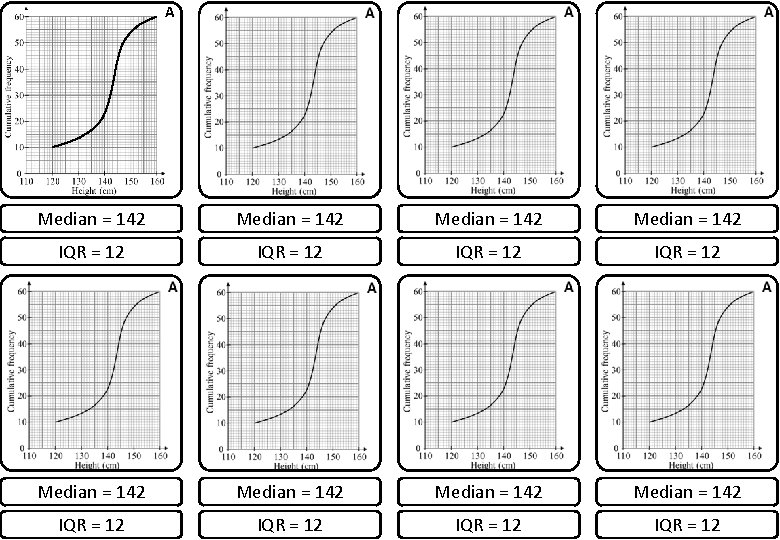A Median = 142 Median = 142 IQR = 12 IQR = 12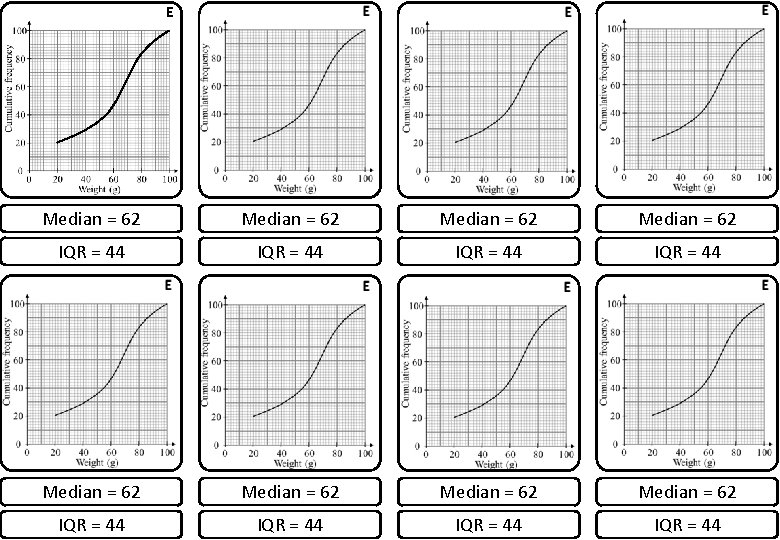E Median = 62 Median = 62 IQR = 44 IQR = 44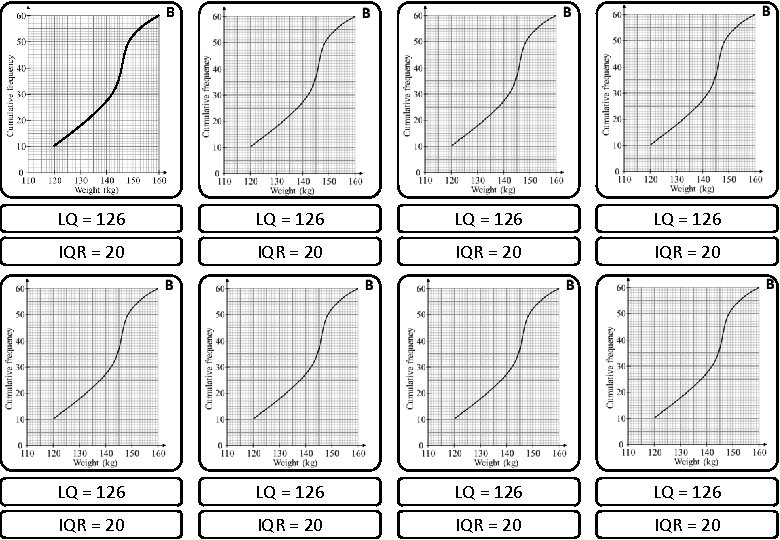B LQ = 126 LQ = 126 IQR = 20 IQR = 20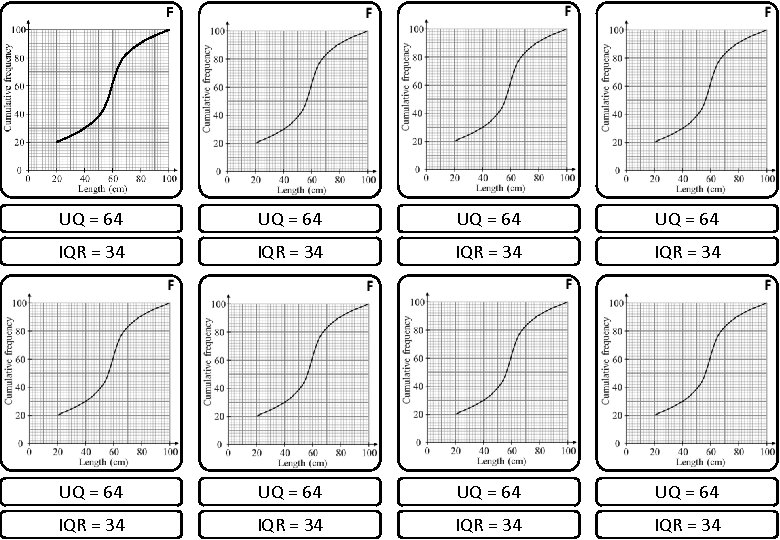F UQ = 64 UQ = 64 IQR = 34 IQR = 34H UQ = 148 UQ = 148 IQR = 14 IQR = 14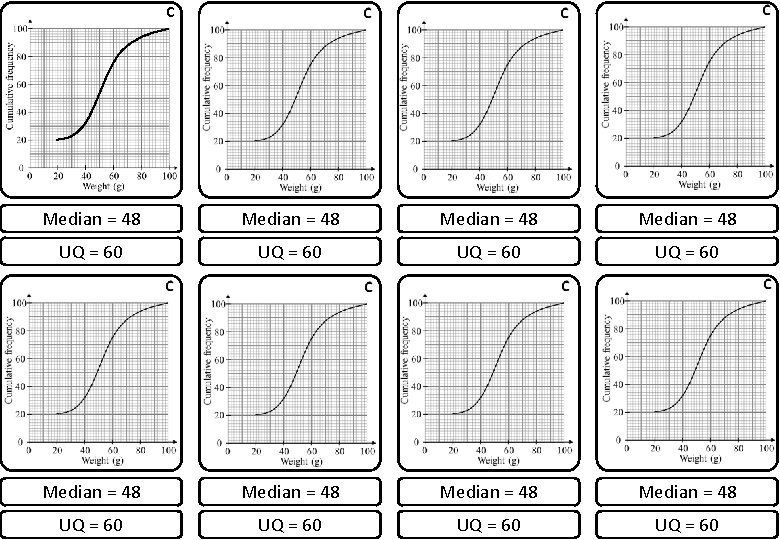C Median = 48 Median = 48 UQ = 60 UQ = 60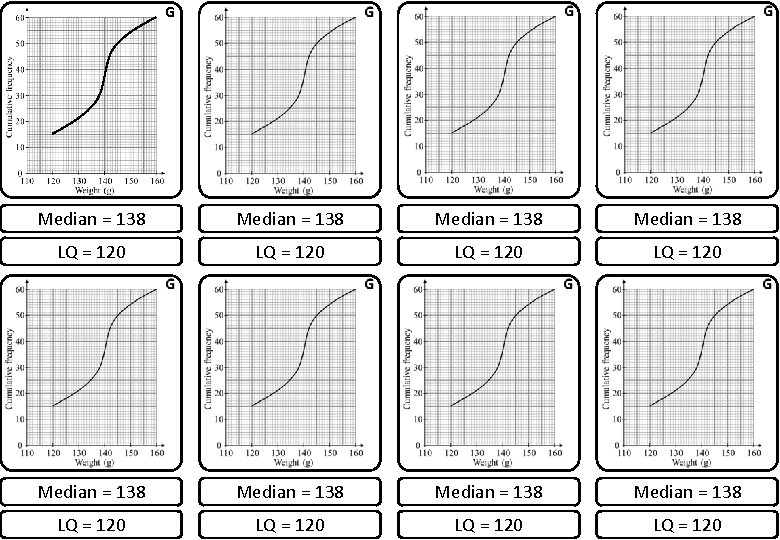G Median = 138 Median = 138 LQ = 120 LQ = 120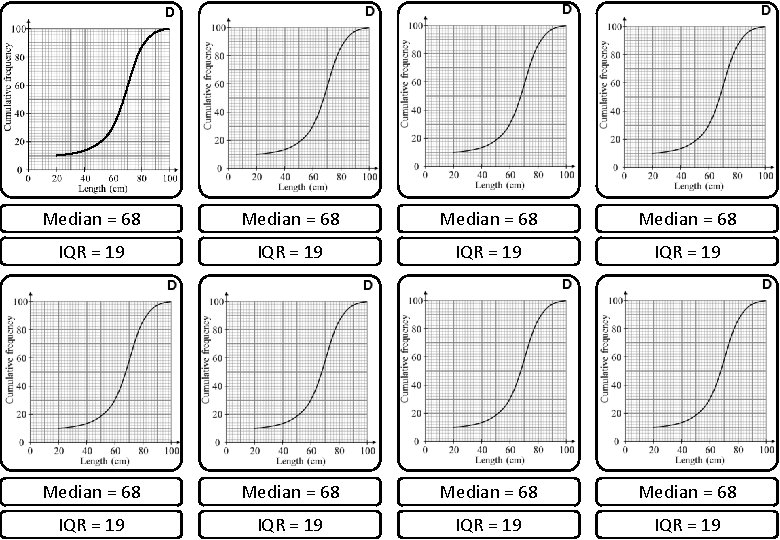D Median = 68 Median = 68 IQR = 19 IQR = 19Questions? Comments? Suggestions? …or have you found a mistake!? Any feedback would be appreciated . Please feel free to email: [email protected] co. uk